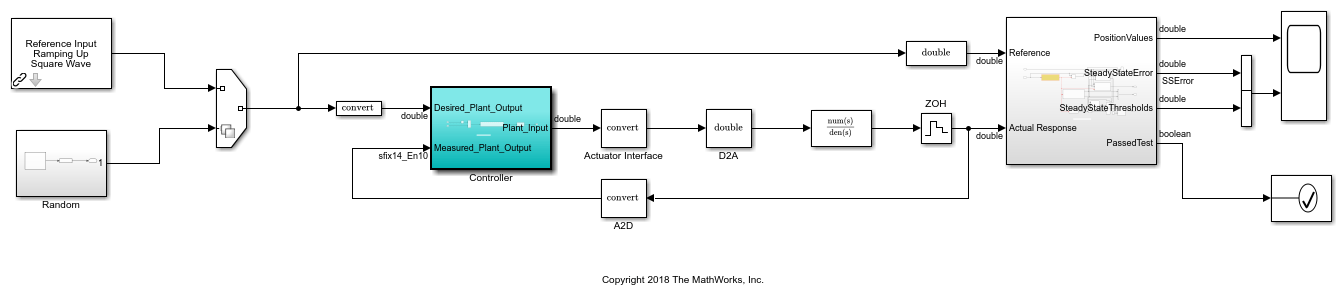## Optimize the Fixed-Point Data Types of a System Using the Fixed-Point Tool

This example shows how to define simulation scenarios and use the Fixed-Point Tool to collect ranges by running simulations using these scenarios. You can then use the Fixed-Point Tool to optimize the fixed-point data types of the system.

### Open Model and Define Simulation Scenarios

Open the model. In this example, you optimize the data types of the Controller subsystem. The model is set up to use either a ramp input, or a random input.

```model = 'ex_controllerHarness'; open_system(model) ```Create a `Simulink.SimulationInput` object that contains the different scenarios. Use both the ramp input as well as four different seeds for the random input.

```si = Simulink.SimulationInput.empty(5, 0); % scan through 4 different seeds for the random input rng(1); seeds = randi(1e6, [1 4]); for sIndex = 1:length(seeds) si(sIndex) = Simulink.SimulationInput(model); si(sIndex) = si(sIndex).setVariable('SOURCE', 2); % SOURCE == 2 corresponds to the random input si(sIndex) = si(sIndex).setBlockParameter([model '/Random/uniformRandom'], 'Seed', num2str(seeds(sIndex))); % scan through the seeds si(sIndex) = si(sIndex).setUserString(sprintf('random_%i', seeds(sIndex))); end % setting SOURCE == 1 corresponds to the ramp input si(5) = Simulink.SimulationInput(model); si(5) = si(5).setVariable('SOURCE', 1); si(5) = si(5).setUserString('Ramp'); ```

### Prepare System for Conversion

To optimize the data types in the mode, use the Fixed-Point Tool.

1. In the Apps gallery of the `ex_controllerHarness` model, select Fixed-Point Tool.

2. In the Fixed-Point Tool, under New workflow, select `Optimized Fixed-Point Conversion`.

3. Under System Under Design (SUD), select the subsystem for which you want to optimize the data types. In this example, select `Controller`.

4. Under Range Collection Mode, select as the range collection method.

5. Under Simulation Inputs, you can specify `Simulink.SimulationInput` objects to exercise your design over its full operating range. In this example, use the simulation scenarios you defined. Set Simulation Inputs to `si`.

6. You can specify tolerances for any signal in the model with signal logging enabled in the table under .

7. In the toolstrip, click . The Fixed-Point Tool checks the system under design for compatibility with the conversion process and reports any issues found in the model. When possible, the Fixed-Point Tool automatically changes settings that are not compatible. For more information, see Use the Fixed-Point Tool to Prepare a System for Conversion.

### Optimize Data Types in the Fixed-Point Tool

1. To specify settings to use during the optimization, in the toolstrip, click .

In this example, use the following settings.

• Set Allowable Word Lengths to `[2:32]`.

This setting defines the word lengths that can be used in your optimized system. Use this setting to target the neighborhood search of the optimization process. The final result of the optimization uses word lengths in the intersection of this setting and word lengths compatible with hardware constraints specified in the Hardware Implementation pane of your model.

• Set Max Iterations to `3e2`.

This setting specifies the maximum number of iterations to perform in the optimization. The optimization process iterates through different solutions until it finds an ideal solution, reaches the maximum number of iterations, or reaches another stopping criteria.

• Set Patience to `50`.

This setting defines the maximum number of iterations where no new best solution is found. The optimization continues as long as the algorithm continues to find new best solutions.

For more information about optimization settings, see `fxpOptimizationOptions`.

2. To optimize the data types in the model according to the specified settings, click . During the optimization process, the software analyzes ranges of objects in your system under design and the constraints specified in the settings to apply heterogeneous data types to your system while minimizing total bit width.

### Examine Results

When the optimization completes, the Fixed-Point Tool displays a table that contains all of the solutions found during the optimization process. The first solution in the table corresponds to the solution with the lowest cost (smallest total bit width).1. To apply the optimized data types to the model, in the table, select the solution that you want to apply. In the Explore section of the toolstrip, click . The Fixed-Point Tool applies the selected solution that contains optimized fixed-point data types to the model and opens the Simulation Data Inspector.

In this example, select Solution 1, then click .

2. In the Controller subsystem, you can see the applied, optimized fixed-point data types.